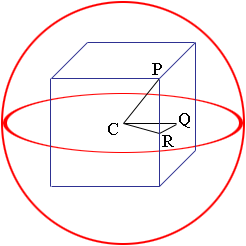Quandaries and Queries Name: Damian Who is asking: Student Level of the question: All Question: A sphere passes through the eight corners of a cube side 10cm. Find the volume of the sphere. Hi Damian, I drew a diagram of the cube inscribed in the sphere.C is the centre of the sphere, R is the midpoint of one of the sides and Q is a midpoint of a face. Since the cube has sides of length 10 cm the length of CQ is 5 cm and the length of RQ is 5 cm. Triangle CRQ is a right triangle so you can use Pythagoras theorem to find the length of CR. Now the triangle PCR is also a right triangle, the length of CR you just found and the length of PR is 5 cm so again you can use Pythagoras theorem, this time to find the length of CP which is the radius of the sphere. Penny